Next: Application: Superdense coding Up: A Quantum Computing Tutor Previous: A Quantum Computing Tutor   Contents

## Qubits - Superposition and Entanglement

Modern classical computers store and manipulate a unit of information called the bit, which takes either 0 or 1 as it's value. The classical paradigm seems to be limited however in the types of problems it can solve; there is a vast amount of problems that are intractable on modern computers. Herein lies the promise of Quantum Computers. A Quantum Computer uses a different fundamental unit of information, called the Qubit. A Qubit has two base states, normally denoted by the Dirac notation,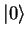and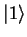. The difference between a qubit and a classical bit, is that it is possible for a qubit to be in a linear combination of states, denoted by;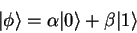(3)

The numbers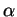and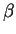are complex numbers. The property of being able to exist in multiple states is called superposition. Quantum mechanics does not allow us to view what the amplitudes, ie.and, of the two basevectors are. Instead, when we measure a qubit, we get the statewith probability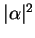, and the statewith probability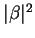. Both of these probabilities must add up to 1. If a quantum operation is performed on a qubit in multiple states, then the operation is performed on all states simultaneously. When the qubit is observed, it will collapse back into a single state stochastically, according to the squares of the probabilities.

The famous physicist R. Feynmann suggested that a qubit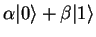occupies all the states between 0 and 1 simultaneously, but collapses into 0 or 1 when observed physically. A qubit can therefore encode an infinite amount of information, but most of this information is useless as it can never be observed. This raises all kinds of interesting philosophical questions about what information is. As stated previously, when observed, a qubit collapses into one of it's basis states. No one knows why this happens, it is one of the basic tenets of quantum mechanics. The explanation that a quantum state collapses upon physical observation is known as the Copenhagen Interpretation, and is the standard(though not the only) way of explaining the collapse upon measurement.

Superposition is one of the properties that allows the Quantum Computing paradigm to supercede classical computing. The other is entanglement. A two qubit system has four computational basis states, which are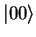,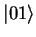,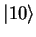and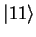. The two qubit system can be in any superposition of these states. There are four very interesting states that such a system can be prepared in. These states are refered to as the Bell States or EPR states. An example of one of these states is the following;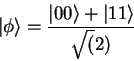(4)

When one measures the first qubit in this state, there are two possible results; 0 with probability 1/2 leaving the other qubit in the state, and 1 with probability 1/2, leaving the other qubit in the state. This means that when the second qubit is measured it will always be in the same state as the first qubit! This correlation between the qubits is known as entanglement. Bell proved that the measurement correlations between the two qubits are stronger than could ever exist between classical systems. For example, entanglement between two qubits can persist even thought they are spatially seperated. Bell states are used as the basis of quantum teleportation and super-dense coding.

The properties of entanglement and superposition mean that certain speedups can be used in quantum algorithms that will never be achievable on classical computers. The two most famous quantum algorithms are Shor's algorithm, which can factor a number which is the product of two large primes in exponential time, and Grover's algorithm which searches an unsorted database in quadratic time.Next: Application: Superdense coding Up: A Quantum Computing Tutor Previous: A Quantum Computing Tutor   Contents
Colm O hEigeartaigh 2003-05-30